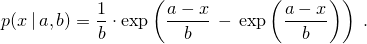# 26 Numerics library [numerics]

## 26.5 Random number generation [rand]

### 26.5.8 Random number distribution class templates [rand.dist]

#### 26.5.8.4.5 Class template extreme_value_distribution[rand.dist.pois.extreme]

An extreme_value_distribution random number distribution produces random numbers x distributed according to the probability density function280template<class RealType = double>
class extreme_value_distribution{
public:
// types
typedef RealType result_type;
typedef unspecified param_type;

// constructor and reset functions
explicit extreme_value_distribution(RealType a = 0.0, RealType b = 1.0);
explicit extreme_value_distribution(const param_type& parm);
void reset();

// generating functions
template<class URNG>
result_type operator()(URNG& g);
template<class URNG>
result_type operator()(URNG& g, const param_type& parm);

// property functions
RealType a() const;
RealType b() const;
param_type param() const;
void param(const param_type& parm);
result_type min() const;
result_type max() const;
};


 explicit extreme_value_distribution(RealType a = 0.0, RealType b = 1.0); 

Requires: 0 < b .

Effects: Constructs an extreme_value_distribution object; a and b correspond to the respective parameters of the distribution.

 RealType a() const; 

Returns: The value of the a parameter with which the object was constructed.

 RealType b() const; 

Returns: The value of the b parameter with which the object was constructed.

The distribution corresponding to this probability density function is also known (with a possible change of variable) as the Gumbel Type I, the log-Weibull, or the Fisher-Tippett Type I distribution.